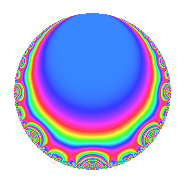Properties

 Label 8016.2.a.nLevel 8016 Weight 2 Character orbit 8016.a Self dual Yes Analytic conductor 64.008 Analytic rank 0 Dimension 3 CM No Inner twists 1

Related objects

Newspace parameters

 Level: $$N$$ = $$8016 = 2^{4} \cdot 3 \cdot 167$$ Weight: $$k$$ = $$2$$ Character orbit: $$[\chi]$$ = 8016.a (trivial)

Newform invariants

 Self dual: Yes Analytic conductor: $$64.0080822603$$ Analytic rank: $$0$$ Dimension: $$3$$ Coefficient field: 3.3.148.1 Coefficient ring: $$\Z[a_1, \ldots, a_{5}]$$ Coefficient ring index: $$1$$ Fricke sign: $$-1$$ Sato-Tate group: $\mathrm{SU}(2)$

$q$-expansion

Coefficients of the $$q$$-expansion are expressed in terms of a basis $$1,\beta_1,\beta_2$$ for the coefficient ring described below. We also show the integral $$q$$-expansion of the trace form.

 $$f(q)$$ $$=$$ $$q$$ $$+ q^{3}$$ $$+ ( 2 - \beta_{2} ) q^{5}$$ $$+ ( 2 - 2 \beta_{1} ) q^{7}$$ $$+ q^{9}$$ $$+O(q^{10})$$ $$q$$ $$+ q^{3}$$ $$+ ( 2 - \beta_{2} ) q^{5}$$ $$+ ( 2 - 2 \beta_{1} ) q^{7}$$ $$+ q^{9}$$ $$+ ( 1 + \beta_{1} - \beta_{2} ) q^{11}$$ $$+ 2 q^{13}$$ $$+ ( 2 - \beta_{2} ) q^{15}$$ $$+ 3 \beta_{2} q^{17}$$ $$+ ( -2 + 2 \beta_{1} + 2 \beta_{2} ) q^{19}$$ $$+ ( 2 - 2 \beta_{1} ) q^{21}$$ $$+ 8 q^{23}$$ $$+ ( 2 - \beta_{1} - 5 \beta_{2} ) q^{25}$$ $$+ q^{27}$$ $$+ ( -4 - 2 \beta_{1} + 2 \beta_{2} ) q^{29}$$ $$+ ( 2 - 2 \beta_{1} - 2 \beta_{2} ) q^{31}$$ $$+ ( 1 + \beta_{1} - \beta_{2} ) q^{33}$$ $$+ ( 2 - 2 \beta_{1} - 2 \beta_{2} ) q^{35}$$ $$+ ( -4 + 2 \beta_{1} ) q^{37}$$ $$+ 2 q^{39}$$ $$+ ( 6 - 6 \beta_{1} - 3 \beta_{2} ) q^{41}$$ $$+ ( 2 + 2 \beta_{1} + 3 \beta_{2} ) q^{43}$$ $$+ ( 2 - \beta_{2} ) q^{45}$$ $$+ ( -1 + 3 \beta_{1} - 3 \beta_{2} ) q^{47}$$ $$+ ( 5 - 4 \beta_{1} + 4 \beta_{2} ) q^{49}$$ $$+ 3 \beta_{2} q^{51}$$ $$+ ( -4 + 4 \beta_{1} + 3 \beta_{2} ) q^{53}$$ $$+ ( 6 - 4 \beta_{2} ) q^{55}$$ $$+ ( -2 + 2 \beta_{1} + 2 \beta_{2} ) q^{57}$$ $$+ ( -2 + 2 \beta_{1} - 2 \beta_{2} ) q^{59}$$ $$+ ( -1 + 5 \beta_{1} + 5 \beta_{2} ) q^{61}$$ $$+ ( 2 - 2 \beta_{1} ) q^{63}$$ $$+ ( 4 - 2 \beta_{2} ) q^{65}$$ $$+ ( 8 + 2 \beta_{1} + \beta_{2} ) q^{67}$$ $$+ 8 q^{69}$$ $$+ ( 4 + 4 \beta_{1} + 6 \beta_{2} ) q^{71}$$ $$+ ( 8 - 2 \beta_{1} - 4 \beta_{2} ) q^{73}$$ $$+ ( 2 - \beta_{1} - 5 \beta_{2} ) q^{75}$$ $$+ ( -4 - 4 \beta_{2} ) q^{77}$$ $$+ ( -4 + 2 \beta_{1} - 5 \beta_{2} ) q^{79}$$ $$+ q^{81}$$ $$+ ( 4 - 8 \beta_{1} - 6 \beta_{2} ) q^{83}$$ $$+ ( -9 + 3 \beta_{1} + 9 \beta_{2} ) q^{85}$$ $$+ ( -4 - 2 \beta_{1} + 2 \beta_{2} ) q^{87}$$ $$+ ( -6 \beta_{1} + 2 \beta_{2} ) q^{89}$$ $$+ ( 4 - 4 \beta_{1} ) q^{91}$$ $$+ ( 2 - 2 \beta_{1} - 2 \beta_{2} ) q^{93}$$ $$+ ( -8 + 4 \beta_{1} + 8 \beta_{2} ) q^{95}$$ $$+ ( 2 - 6 \beta_{2} ) q^{97}$$ $$+ ( 1 + \beta_{1} - \beta_{2} ) q^{99}$$ $$+O(q^{100})$$ $$\operatorname{Tr}(f)(q)$$ $$=$$ $$3q$$ $$\mathstrut +\mathstrut 3q^{3}$$ $$\mathstrut +\mathstrut 6q^{5}$$ $$\mathstrut +\mathstrut 4q^{7}$$ $$\mathstrut +\mathstrut 3q^{9}$$ $$\mathstrut +\mathstrut O(q^{10})$$ $$3q$$ $$\mathstrut +\mathstrut 3q^{3}$$ $$\mathstrut +\mathstrut 6q^{5}$$ $$\mathstrut +\mathstrut 4q^{7}$$ $$\mathstrut +\mathstrut 3q^{9}$$ $$\mathstrut +\mathstrut 4q^{11}$$ $$\mathstrut +\mathstrut 6q^{13}$$ $$\mathstrut +\mathstrut 6q^{15}$$ $$\mathstrut -\mathstrut 4q^{19}$$ $$\mathstrut +\mathstrut 4q^{21}$$ $$\mathstrut +\mathstrut 24q^{23}$$ $$\mathstrut +\mathstrut 5q^{25}$$ $$\mathstrut +\mathstrut 3q^{27}$$ $$\mathstrut -\mathstrut 14q^{29}$$ $$\mathstrut +\mathstrut 4q^{31}$$ $$\mathstrut +\mathstrut 4q^{33}$$ $$\mathstrut +\mathstrut 4q^{35}$$ $$\mathstrut -\mathstrut 10q^{37}$$ $$\mathstrut +\mathstrut 6q^{39}$$ $$\mathstrut +\mathstrut 12q^{41}$$ $$\mathstrut +\mathstrut 8q^{43}$$ $$\mathstrut +\mathstrut 6q^{45}$$ $$\mathstrut +\mathstrut 11q^{49}$$ $$\mathstrut -\mathstrut 8q^{53}$$ $$\mathstrut +\mathstrut 18q^{55}$$ $$\mathstrut -\mathstrut 4q^{57}$$ $$\mathstrut -\mathstrut 4q^{59}$$ $$\mathstrut +\mathstrut 2q^{61}$$ $$\mathstrut +\mathstrut 4q^{63}$$ $$\mathstrut +\mathstrut 12q^{65}$$ $$\mathstrut +\mathstrut 26q^{67}$$ $$\mathstrut +\mathstrut 24q^{69}$$ $$\mathstrut +\mathstrut 16q^{71}$$ $$\mathstrut +\mathstrut 22q^{73}$$ $$\mathstrut +\mathstrut 5q^{75}$$ $$\mathstrut -\mathstrut 12q^{77}$$ $$\mathstrut -\mathstrut 10q^{79}$$ $$\mathstrut +\mathstrut 3q^{81}$$ $$\mathstrut +\mathstrut 4q^{83}$$ $$\mathstrut -\mathstrut 24q^{85}$$ $$\mathstrut -\mathstrut 14q^{87}$$ $$\mathstrut -\mathstrut 6q^{89}$$ $$\mathstrut +\mathstrut 8q^{91}$$ $$\mathstrut +\mathstrut 4q^{93}$$ $$\mathstrut -\mathstrut 20q^{95}$$ $$\mathstrut +\mathstrut 6q^{97}$$ $$\mathstrut +\mathstrut 4q^{99}$$ $$\mathstrut +\mathstrut O(q^{100})$$

Basis of coefficient ring in terms of a root $$\nu$$ of $$x^{3}\mathstrut -\mathstrut$$ $$x^{2}\mathstrut -\mathstrut$$ $$3$$ $$x\mathstrut +\mathstrut$$ $$1$$:

 $$\beta_{0}$$ $$=$$ $$1$$ $$\beta_{1}$$ $$=$$ $$\nu$$ $$\beta_{2}$$ $$=$$ $$\nu^{2} - \nu - 2$$
 $$1$$ $$=$$ $$\beta_0$$ $$\nu$$ $$=$$ $$\beta_{1}$$ $$\nu^{2}$$ $$=$$ $$\beta_{2}\mathstrut +\mathstrut$$ $$\beta_{1}\mathstrut +\mathstrut$$ $$2$$

Embeddings

For each embedding $$\iota_m$$ of the coefficient field, the values $$\iota_m(a_n)$$ are shown below.

For more information on an embedded modular form you can click on its label.

Label $$\iota_m(\nu)$$ $$a_{2}$$ $$a_{3}$$ $$a_{4}$$ $$a_{5}$$ $$a_{6}$$ $$a_{7}$$ $$a_{8}$$ $$a_{9}$$ $$a_{10}$$
1.1
 −1.48119 2.17009 0.311108
0 1.00000 0 0.324869 0 4.96239 0 1.00000 0
1.2 0 1.00000 0 1.46081 0 −2.34017 0 1.00000 0
1.3 0 1.00000 0 4.21432 0 1.37778 0 1.00000 0
 $$n$$: e.g. 2-40 or 990-1000 Significant digits: Format: Complex embeddings Normalized embeddings Satake parameters Satake angles

Inner twists

This newform does not admit any (nontrivial) inner twists.

Atkin-Lehner signs

$$p$$ Sign
$$2$$ $$1$$
$$3$$ $$-1$$
$$167$$ $$1$$

Hecke kernels

This newform can be constructed as the intersection of the kernels of the following linear operators acting on $$S_{2}^{\mathrm{new}}(\Gamma_0(8016))$$:

 $$T_{5}^{3}$$ $$\mathstrut -\mathstrut 6 T_{5}^{2}$$ $$\mathstrut +\mathstrut 8 T_{5}$$ $$\mathstrut -\mathstrut 2$$ $$T_{7}^{3}$$ $$\mathstrut -\mathstrut 4 T_{7}^{2}$$ $$\mathstrut -\mathstrut 8 T_{7}$$ $$\mathstrut +\mathstrut 16$$ $$T_{11}^{3}$$ $$\mathstrut -\mathstrut 4 T_{11}^{2}$$ $$\mathstrut -\mathstrut 4 T_{11}$$ $$\mathstrut +\mathstrut 20$$ $$T_{13}$$ $$\mathstrut -\mathstrut 2$$